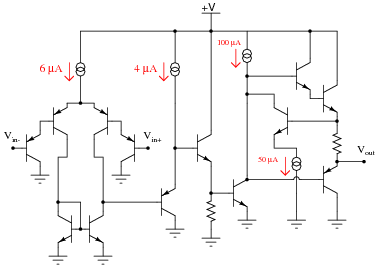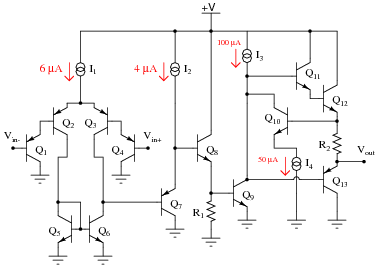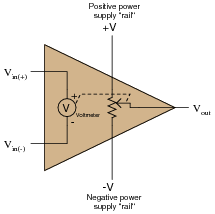# Basic Operational Amplifiers

• #### Question 1

Determine the output voltage polarity of this op-amp (with reference to ground), given the following input conditions:• #### Question 2

 Don’t just sit there! Build something!!

Learning to mathematically analyze circuits requires much study and practice. Typically, students practice by working through lots of sample problems and checking their answers against those provided by the textbook or the instructor. While this is good, there is a much better way.

You will learn much more by actually building and analyzing real circuits, letting your test equipment provide the “answers” instead of a book or another person. For successful circuit-building exercises, follow these steps:

1. Carefully measure and record all component values prior to circuit construction.
2. Draw the schematic diagram for the circuit to be analyzed.
3. Carefully build this circuit on a breadboard or other convenient medium.
4. Check the accuracy of the circuit’s construction, following each wire to each connection point, and verifying these elements one-by-one on the diagram.
5. Mathematically analyze the circuit, solving for all voltage and current values.
6. Carefully measure all voltages and currents, to verify the accuracy of your analysis.
7. If there are any substantial errors (greater than a few percent), carefully check your circuit’s construction against the diagram, then carefully re-calculate the values and re-measure.

Avoid using the model 741 op-amp, unless you want to challenge your circuit design skills. There are more versatile op-amp models commonly available for the beginner. I recommend the LM324 for DC and low-frequency AC circuits, and the TL082 for AC projects involving audio or higher frequencies.

As usual, avoid very high and very low resistor values, to avoid measurement errors caused by meter “loading”. I recommend resistor values between 1 kΩ and 100 kΩ.

One way you can save time and reduce the possibility of error is to begin with a very simple circuit and incrementally add components to increase its complexity after each analysis, rather than building a whole new circuit for each practice problem. Another time-saving technique is to re-use the same components in a variety of different circuit configurations. This way, you won’t have to measure any component’s value more than once.

• #### Question 3

An operational amplifier is a particular type of differential amplifier. Most op-amps receive two input voltage signals and output one voltage signal:Here is a single op-amp, shown under two different conditions (different input voltages). Determine the voltage gain of this op-amp, given the conditions shown:Also, write a mathematical formula solving for differential voltage gain (AV) in terms of an op-amp’s input and output voltages.

• #### Question 4

Many op-amp circuits require a dual or split power supply, consisting of three power terminals: V, -V, and Ground. Draw the necessary connections between the 6-volt batteries in this schematic diagram to provide 12 V, -12 V, and Ground to this op-amp:• #### Question 5

The 8-pin Dual-Inline-Package (DIP) is a common format in which single and dual operational amplifiers are housed. Shown here are the case outlines for two 8-pin DIPs. Draw the internal op-amp connections for a single op-amp unit, and for a dual op-amp unit:You will need to research some op-amp datasheets to find this information. Examples of single op-amp chips include the LM741, CA3130, and TL081. Examples of dual op-amp chips include the LM1458 and TL082.

• #### Question 6

Shown here is a simplified schematic diagram of one of the operational amplifiers inside a TL08x (TL081, TL082, or TL084) op-amp integrated circuit:Qualitatively determine what will happen to the output voltage (Vout) if the voltage on the noninverting input (Vin ) increases, and the voltage on the inverting input (Vin−) remains the same (all voltages are positive quantities, referenced to -V). Explain what happens at every stage of the op-amp circuit (voltages increasing or decreasing, currents increasing or decreasing) with this change in input voltage.

• #### Question 7

Shown here is a simplified schematic diagram of one of the operational amplifiers inside an LM324 quad op-amp integrated circuit:Qualitatively determine what will happen to the output voltage (Vout) if the voltage on the inverting input (Vin−) increases, and the voltage on the non-inverting input (Vin ) remains the same (all voltages are positive quantities, referenced to ground). Explain what happens at every stage of the op-amp circuit (voltages increasing or decreasing, currents increasing or decreasing) with this change in input voltage.

• #### Question 8

One of the first popular operational amplifiers was manufactured by Philbrick Researches, and it was called the K2-W. Built with two dual-triode vacuum tubes, its original schematic diagram looked like this:To make this opamp circuit easier for modern students to understand, I’ll substitute equivalent solid-state components for all tubes in the original design:Explain the configuration (common-source, common-drain, or common-gate) of each transistor in the modernized schematic, identifying the function of each in the operational amplifier circuit.

• #### Question 9

Determine the functions for as many components as you can in the following schematic diagram of a model 741 operational amplifier:• #### Question 10

Predict how the operation of this operational amplifier circuit will be affected as a result of the following faults. Specifically, identify whether the output voltage (Vout) will move in a positive direction (closer to the V rail) or in a negative direction (closer to ground). Consider each fault independently (i.e. one at a time, no multiple faults):Transistor Q5 fails shorted (collector-to-emitter):
Transistor Q6 fails shorted (collector-to-emitter):
Resistor R1 fails open:
Current source I2 fails shorted:

For each of these conditions, explain why the resulting effects will occur.

• #### Question 11

Predict how the operation of this operational amplifier circuit will be affected as a result of the following faults. Specifically, identify whether the output voltage (Vout) will move in a positive direction (closer to the V rail) or in a negative direction (closer to the -V rail). Consider each fault independently (i.e. one at a time, no multiple faults):Diode D1 fails open:
Resistor R1 fails shorted:
Transistor Q2 fails shorted (drain-to-source):
Transistor Q5 fails shorted (collector-to-emitter):
Resistor R2 fails open:
Current source I2 fails open:

For each of these conditions, explain why the resulting effects will occur.

• #### Question 12

Ideally, what should the output voltage of an op-amp do if the non-inverting voltage is greater (more positive) than the inverting voltage?• #### Question 13

A helpful model for understanding opamp function is one where the output of an opamp is thought of as being the wiper of a potentiometer, the wiper position automatically adjusted according to the difference in voltage measured between the two inputs:To elaborate further, imagine an extremely sensitive, analog, zero-center voltmeter inside the opamp, where the moving-coil mechanism of the voltmeter mechanically drives the potentiometer wiper. The wiper’s position would then be proportional to both the magnitude and polarity of the difference in voltage between the two input terminals.

Realistically, building such a voltmeter/potentiometer mechanism with the same sensitivity and dynamic performance as a solid-state opamp circuit would be impossible, but the point here is to model the opamp in terms of components that we are already very familiar with, not to suggest an alternative construction for real opamps.

Describe how this model helps to explain the output voltage limits of an opamp, and also where the opamp sources or sinks load current from.

• #### Question 14

In this circuit, an op-amp turns on an LED if the proper input voltage conditions are met:Trace the complete path of current powering the LED. Where, exactly, does the LED get its power from?

• #### Question 15

Ideally, when the two input terminals of an op-amp are shorted together (creating a condition of zero differential voltage), and those two inputs are connected directly to ground (creating a condition of zero common-mode voltage), what should this op-amp’s output voltage be?In reality, the output voltage of an op-amp under these conditions is not the same as what would be ideally predicted. Identify the fundamental problem in real op-amps, and also identify the best solution.

• #### Question 16

What does it mean if an operational amplifier has the ability to “swing its output rail to rail”? Why is this an important feature to us?